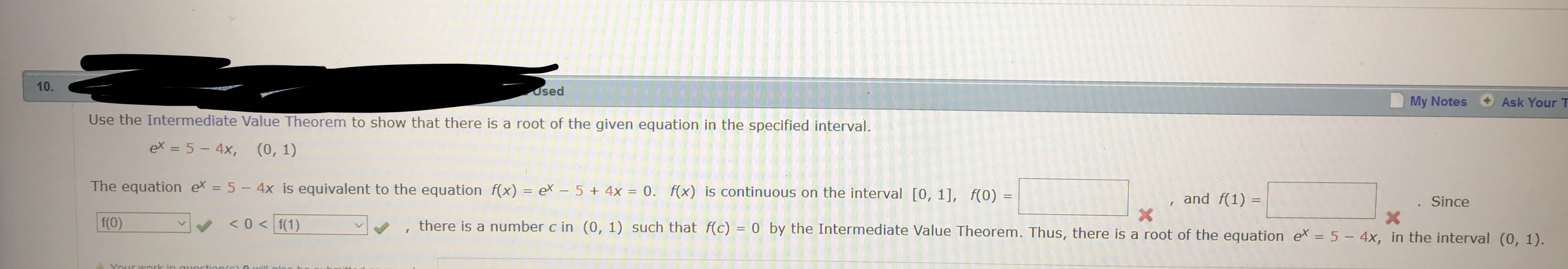# 10osedMy NotesAsk Your TUse the Intermediate Value Theorem to show that there is a root of the given equation in the specified intervalex = 5-4),(0,1)The equation ex-5- 4x is equivalent to the equation f(x) ex 54x 0fx) is continuous on the interval [0, 11, f(0), and f(1)-Sincev,, there is a number c in (0, 1) such that f(c)-0 by the Intermediate Value Theorem. Thus, there is a root of the

Question
24 viewshelp_outlineImage Transcriptionclose10 osed My Notes Ask Your T Use the Intermediate Value Theorem to show that there is a root of the given equation in the specified interval ex = 5-4), (0,1) The equation ex-5- 4x is equivalent to the equation f(x) ex 5 4x 0fx) is continuous on the interval [0, 11, f(0) , and f(1)- Since v, , there is a number c in (0, 1) such that f(c)-0 by the Intermediate Value Theorem. Thus, there is a root of the fullscreen
check_circle

Step 1

We have been given an equation and an interval as shown below:

We can ...

### Want to see the full answer?

See Solution

#### Want to see this answer and more?

Solutions are written by subject experts who are available 24/7. Questions are typically answered within 1 hour.*

See Solution
*Response times may vary by subject and question.
Tagged in

### Continuity